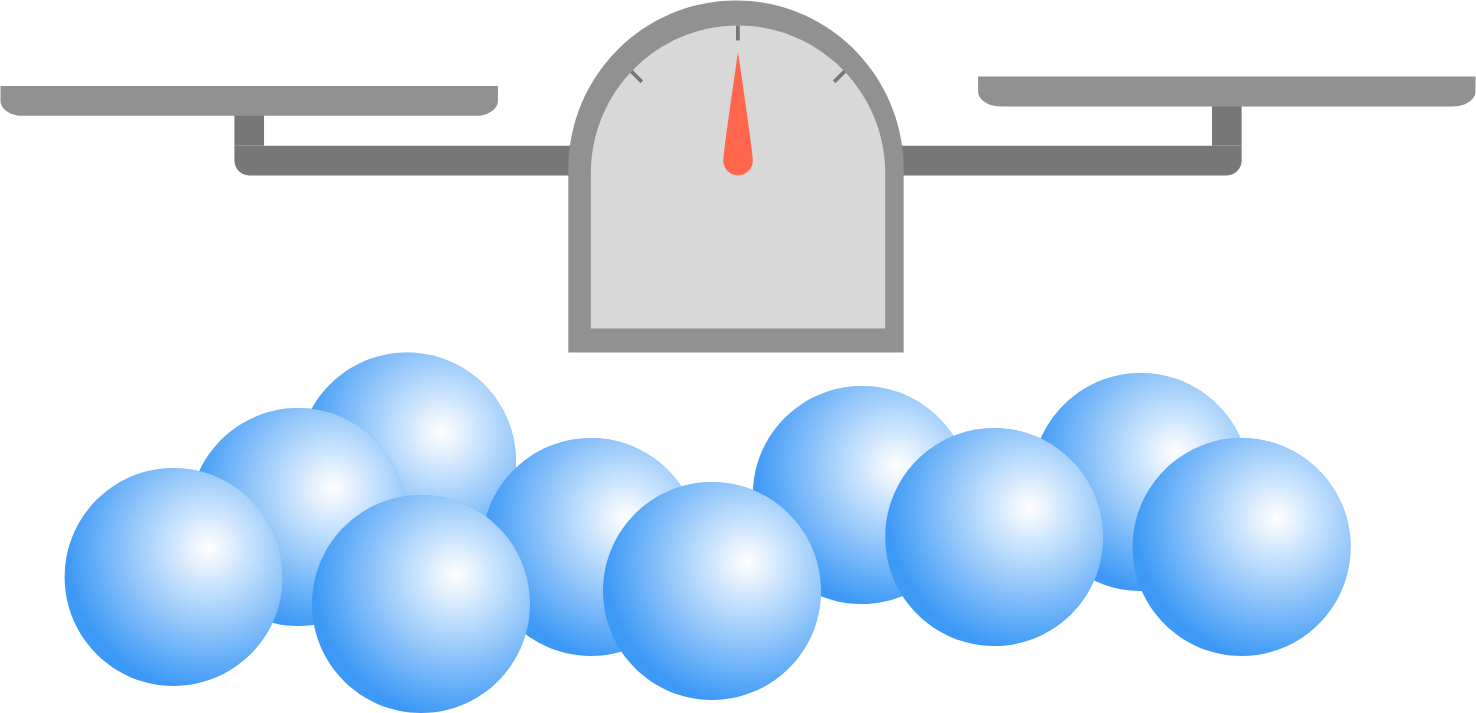# Tous les deux

Logic Level 4

Suppose there are 10 balls identical in appearance, where 8 of them each have a mass of $x$ grams, and each of the other two $(x + \delta)$ grams. Now, $\delta \, (\gt 0)$ is so small that the difference can't be detected using your own hands, and can only be detected by the precise balance scale you have been provided with. You can put any number of balls in each pan of the scale.

What is the minimum number of weighings you will need to make to guarantee the identification of both of the heavier balls?×